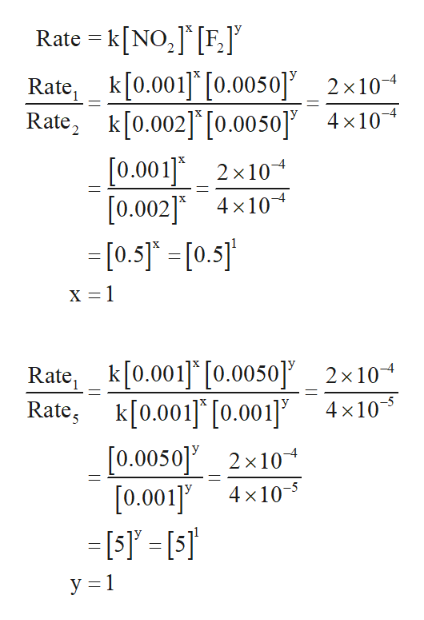# How can I determine the order of the reaction with respect to each reactant? Experiment 1: .0010 M NO2, .0050 M F2; initial rate= 2.0 x 10^-4 molF2/ L x sExperiment 2: .0020 M NO2, .0050 M F2; initial rate= 4.0 x 10^-4 molF2/L x sExperiment 5: .0010 M NO2, .0010 M F2; initial rate= 4.0 x 10^-5 molF2/L x s

Question

How can I determine the order of the reaction with respect to each reactant?

Experiment 1: .0010 M NO2, .0050 M F2; initial rate= 2.0 x 10^-4 molF2/ L x s

Experiment 2: .0020 M NO2, .0050 M F2; initial rate= 4.0 x 10^-4 molF2/L x s

Experiment 5: .0010 M NO2, .0010 M F2; initial rate= 4.0 x 10^-5 molF2/L x s

check_circleExpert Solution
Step 1

The overall order for the given reaction is determined as ...help_outlineImage TranscriptioncloseRate = k[NO,] [F.J Rate, k[0.001 [0.0050]2x10 Rate, k[0.002] [0.0050] 4x10 [0.001] 2x10 [0.002] 4x10 =[0.5] =[0.5] x 1 Rate, k[0.001] [0.0050] _ 2x10 Rate, k[0.001] [0.001] 4x10 [0.0050]2x10 [0.001]4x10- У 31 fullscreen

### Want to see the full answer?

See Solution

#### Want to see this answer and more?

Solutions are written by subject experts who are available 24/7. Questions are typically answered within 1 hour*

See Solution
*Response times may vary by subject and question
Tagged in

### Chemical Kinetics